Q-Chem 5.0 User’s Manual

# 12.11 The XPol+SAPT (XSAPT) Method

## 12.11.1 Theory

XPol+SAPT, or “XSAPT”, was introduced by Jacobson and Herbert [846, 591] as a low-order-scaling, systematically-improvable method applicable to large systems. The idea is simply to replace the need for empirical parameters in the XPol method with on-the-fly evaluation of exchange-repulsion and dispersion interactions via pairwise-additive SAPT. (This basic idea has spawned several related approaches; see Ref. Lao:2015 for an overview of XSAPT-based methods.) Stated differently, XSAPT uses XPol to evaluate many-body (non-pairwise-additive) polarization effects, but then assumes that dispersion and exchange-repulsion interactions are  pairwise additive, and evaluates them via pairwise SAPT0 or SAPT(KS) calculations.

In particular, the zeroth-order Hamiltonian for XSAPT is taken by the sum of fragment Fock operators defined by the XPol procedure, and the perturbation is the usual SAPT intermolecular perturbation [Eq. eq:pairwise_perturbation] less the intermolecular interactions contained in the XPol fragment Fock operators. A standard SAPT0 correction is then computed for each pair of monomers, using Eq. eq:SAPT_interaction in conjunction with the modified perturbation, to obtain dimer interaction energy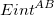. The total XSAPT energy is then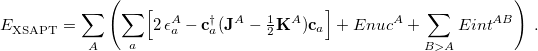(12.38)

In this expression, we have removed the over-counting of two-electron interactions present in Hartree-Fock theory, effectively taking the intrafragment perturbation to first order. The generalization to a Kohn-Sham description of the monomers is straightforward, and is available in Q-Chem.

The inclusion of many-body polarization within the zeroth-order Hamiltonian makes the subsequent SAPT corrections less meaningful in terms of energy decomposition analysis. For instance, the first-order electrostatic correction in XSAPT is not  the total electrostatic energy, since the former corrects for errors in the approximate electrostatic treatment at zeroth order (i.e., the electrostatic embedding). The dispersion correction may be less contaminated, since all of the XSAPT modifications to the traditional SAPT perturbation are one-electron operators and therefore the pairwise dispersion correction differs from its traditional SAPT analogue only insofar as the MOs are perturbed by the electrostatic embedding. This should be kept in mind when interpreting the output of an XSAPT calculation, although Lao and Herbert [851, 852] later proposed a many-body energy decomposition scheme for XSAPT that extends traditional SAPT energy decomposition to systems containing more than two monomers. (The aforementioned contamination problems are avoid through pairwise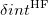corrections, comparing XSAPT results to traditional SAPT based on gas-phase monomers.) An XSAPT calculation is requested by setting the $rem variables XPOL and SAPT equal to TRUE and also setting XPOL_MPOL_ORDER = CHARGES. Researchers who use Q-Chem’s XPol+SAPT code are asked to cite Refs. Jacobson:2011 and Herbert:2012. The latter contains a thorough discussion of the theory; a briefer summary can be found in Ref. Jacobson:2013. Example 12.318 Example showing an XPol+SAPT0 calculation using CHELPG charges and CPHF. $rem
BASIS            CC-PVDZ
METHOD           HF
XPOL             TRUE
XPOL_MPOL_ORDER  CHARGES
XPOL_CHARGE_TYPE QCHELPG
SAPT             TRUE
SAPT_CPHF        TRUE
SYM_IGNORE       TRUE
$end$molecule
0 1
-- formic acid
0 1
C  -1.888896 -0.179692  0.000000
O  -1.493280  1.073689  0.000000
O  -1.170435 -1.166590  0.000000
H  -2.979488 -0.258829  0.000000
H  -0.498833  1.107195  0.000000
-- formic acid
0  1
C  1.888896  0.179692  0.000000
O  1.493280 -1.073689  0.000000
O  1.170435  1.166590  0.000000
H  2.979488  0.258829  0.000000
H  0.498833 -1.107195  0.000000
$end  ## 12.11.2 AO-XSAPT(KS)+D As mentioned above, the dispersion components of the (X)SAPT(KS) interaction energy are not of benchmark quality, even when tuned LRC functionals are employed . The dispersion and exchange-dispersion terms are also the most expensive part of a SAPT0 or SAPT(KS) calculation, scaling as the fourth and fifth powers, respectively, of monomer size, whereas other terms are cubic scaling at worst. Both the efficiency and the accuracy of XSAPT(KS) calculations are thus improved if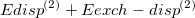is replaced by an empirical atom–atom dispersion potential and those non-dispersion components are reformulated in the atomic orbital (AO) basis to avoid the four-index integral transformation that is required in the original, molecular orbital version of the method. The resulting method is called AO-XSAPT(KS)+D [850, 851, 852]; consult Ref. Lao:2015 for an overview. An AO-XSAPT(KS)+D calculation is requested by setting SAPT_AO and SAPT_DISP_CORR equal to TRUE. There are three versions of the dispersion potential, a “first generation" (+D1) version , second-generation (+D2) version , and third-generation (+D3) version ; the user can select amongst these using SAPT_DISP_VERSION. Although all three versions exhibit similar performance for total binding energies , the +D2 and +D3 potentials were fit directly to ab initio dispersion potentials and do a much better job of reproducing individual energy components, as compared to +D1 [851, 852]. Furthermore, the training set was expanded in the third generation to eliminate the largest errors in +D2 calculations, which tend to be-stacked systems, and +D3 is the recommended dispersion correction. As with XPol, the XSAPT and XSAPT(KS)+D methods do not function with a solvation model or with external changes. Currently, only single-point energies are available with no frozen orbitals. Researchers who use XSAPT(KS)+D are asked to cite Ref. Lao:2012b for +D1, Ref. Lao:2013 for +D2, or Ref. Lao:2015 for +D3. The AO implementation of XSAPT is described in Ref. Lao:2017. SAPT_AO  Request an atomic-orbital version of SAPT TYPE:  BOOLEAN DEFAULT:  FALSE OPTIONS:  TRUE Use an AO version of SAPT. FALSE Use a MO version of SAPT. RECOMMENDATION:  Non-dispersion terms are calculated by AO-SAPT with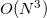scaling. SAPT_DISP_CORR  Request an empirical dispersion potential instead of calculating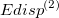and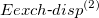directly TYPE:  BOOLEAN DEFAULT:  FALSE OPTIONS:  TRUE Use a dispersion force field. FALSE calculateand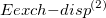. RECOMMENDATION:  Using dispersion potentials combined with AO version of SAPT reduces the scaling from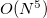towith respect to monomer size. SAPT_DISP_VERSION  Controls which dispersion potential is used for SAPT TYPE:  INTEGER DEFAULT:  3 OPTIONS:  1 Use the “first generation” (+D1) dispersion potentials from Hesselmann [880, 850]. 2 Use the “second generation” (+D2) dispersion potentials from Podeszwa. [881, 851]. 3 Use the “third generation” (+D3) dispersion potentials from Lao . RECOMMENDATION:  Use +D3. Whereas +D1 was fit to reproduce binding energies, the +D2 and +D3 potentials were fit directly to dispersion energies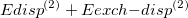computed at the SAPT(DFT) and SAPT2+(3) levels, and performs well for both total binding energies as well as individual energy components [851, 852]. In developing +D3, the training set was expanded to eliminate outliers involvingstacking . Example 12.319 AO-XSAPT(KS)+D3 calculation of water-water interaction. $rem
SYM_IGNORE         true
EXCHANGE           gen
BASIS              aug-cc-pVTZ
XPOL               true ! must be set to true for sapt jobs too
XPOL_MPOL_ORDER    charges
XPOL_CHARGE_TYPE   qchelpg
XPOL_OMEGA         true
XPOL_PRINT         3
SAPT_PRINT         3
SAPT               true
SAPT_AO            true
SAPT_ORDER         2          ! can be set to 1, ELST or RSPT
SAPT_BASIS         projected ! monomer, dimer (if only 2 monomers), or projected
SAPT_DISP_CORR     true
SAPT_DISP_VERSION  3
MEM_TOTAL          46000
MEM_STATIC         4000
AO2MO_DISK         35000
THRESH             12
SCF_CONVERGENCE    7
LRC_DFT            true
CHELPG             true
CHELPG_DX          5
CHELPG_H           110
CHELPG_HA          590
$end$xc_functional
x   wPBE   1.0
c   PBE   1.0
$end$lrc_omega
502
502
$end$molecule
0 1
--
0 1
O  -1.551007  -0.114520   0.000000
H  -1.934259   0.762503   0.000000
H  -0.599677   0.040712   0.000000
--
0 1
O   1.350625   0.111469   0.000000
H   1.680398  -0.373741  -0.758561
H   1.680398  -0.373741   0.758561
$end  For energy decomposition analysis using, e.g., AO-XSAPT(KS)+D3, three individual calculations must be performed: AO-XSAPT(KS)+D3, AO-SAPT(KS)+D3, and AO-SAPT0, as demonstrated in the example below. The electrostatic, exchange, and dispersion energies can be obtained in the AO-SAPT(KS)+D3 calculation by searching the keywords “E1_elst”, “E1_exch”, and “Empirical E2_disp + E2_exch-disp”, respectively. The induction energy includes three parts. First, there is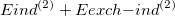(“E2_ind” and “E2_exch-ind”), obtained from the AO-SAPT(KS)+D3 calculation. The second part is the total energy difference between AO-XSAPT(KS)+D3 and AO-SAPT(KS)+D3, and the total energy can be obtained by searching for “SAPT corrected total energy” in both calculations. This second part provides the many-body polarization and approximate infinite-order response correction. The final part of the energy decomposition accounts for higher-order polarization effects that can be approximated by searching the AO-SAPT0 calculation for the keyword “dSCF” (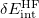correction). The total binding energy is the sum of electrostatic, exchange, induction, and dispersion components obtained above. Example 12.320 The AO-XSAPT(KS)+D3 energy-decomposition analysis for water dimer, which requires three individual calculations. $comment
(1) AO-XSAPT(KS)+D3
$end$rem
SYM_IGNORE         true
EXCHANGE           gen
BASIS              cc-pVDZ
XPOL               true ! must be set to true for sapt jobs too
XPOL_MPOL_ORDER    charges ! gas or charges
XPOL_CHARGE_TYPE   qchelpg  ! qlowdin,qmulliken,qchelpg
XPOL_OMEGA         true
XPOL_PRINT         3
SAPT_PRINT         3
SAPT               true
SAPT_AO            true
SAPT_ORDER         2          ! can be set to 1, ELST or RSPT
SAPT_BASIS         projected ! monomer, dimer (if only 2 monomers), or projected
SAPT_DISP_CORR     true
SAPT_DISP_VERSION  3
MEM_TOTAL          46000
MEM_STATIC         4000
AO2MO_DISK         35000
THRESH             12
SCF_CONVERGENCE    7
LRC_DFT            true
CHELPG             true
CHELPG_DX          5
CHELPG_H           110
CHELPG_HA          590
$end$xc_functional
x   wPBE   1.0
c    PBE   1.0
$end$lrc_omega
502
502
$end$molecule
0 1
--
0 1
O  -1.551007  -0.114520   0.000000
H  -1.934259   0.762503   0.000000
H  -0.599677   0.040712   0.000000
--
0 1
O   1.350625   0.111469   0.000000
H   1.680398  -0.373741  -0.758561
H   1.680398  -0.373741   0.758561
$end @@@$comment
(2) AO-SAPT(KS)+D3
$end$rem
SYM_IGNORE         true
EXCHANGE           gen
BASIS              cc-pVDZ
XPOL               true ! must be set to true for sapt jobs too
XPOL_MPOL_ORDER    gas ! gas or charges
XPOL_OMEGA         true
XPOL_PRINT         3
SAPT_PRINT         3
SAPT               true
SAPT_AO            true
SAPT_ORDER         2          ! can be set to 1, ELST or RSPT
SAPT_BASIS         projected ! monomer, dimer (if only 2 monomers), or projected
SAPT_DISP_CORR     true
SAPT_DISP_VERSION  3
MEM_TOTAL          46000
MEM_STATIC         4000
AO2MO_DISK         35000
THRESH             12
SCF_CONVERGENCE    7
LRC_DFT            true
$end$xc_functional
x   wPBE   1.0
c    PBE   1.0
$end$lrc_omega
502
502
$end$molecule
0 1
--
0 1
O  -1.551007  -0.114520   0.000000
H  -1.934259   0.762503   0.000000
H  -0.599677   0.040712   0.000000
--
0 1
O   1.350625   0.111469   0.000000
H   1.680398  -0.373741  -0.758561
H   1.680398  -0.373741   0.758561
$end @@@$comment
(3) AO-SAPT0
$end$rem
SYM_IGNORE        true
EXCHANGE          hf
BASIS             cc-pVDZ
XPOL              true ! must be set to true for sapt jobs too
XPOL_MPOL_ORDER   gas ! gas or charges
XPOL_PRINT        3
SAPT_PRINT        3
SAPT              true
SAPT_AO           true
SAPT_ORDER        2          ! can be set to 1, ELST or RSPT
SAPT_BASIS        dimer ! monomer, dimer (if only 2 monomers), or projected
SAPT_CPHF         true
SAPT_DSCF         true
MEM_TOTAL         46000
MEM_STATIC        4000
AO2MO_DISK        35000
THRESH            12
SCF_CONVERGENCE   7
$end$molecule
0 1
--
0 1
O  -1.551007  -0.114520   0.000000
H  -1.934259   0.762503   0.000000
H  -0.599677   0.040712   0.000000
--
0 1
O   1.350625   0.111469   0.000000
H   1.680398  -0.373741  -0.758561
H   1.680398  -0.373741   0.758561
\$end